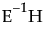Response Specification Panel
 Choose Response Univariate Tests Also Obtains adjusted and unadjusted univariate repeated measures tests and multivariate tests. Use in repeated measures models. Test Each Column Separately Also Obtains univariate ANOVA tests and multivariate tests on each response. The following buttons only appear once you have chosen a response option: Run Help Takes you to the help for the Response Specification panel. Orthogonalize Orthonormalizes the matrix. Orthonormalization is done after the column contrasts (sum to zero) for all response types except Sum. Delete Last Column Reduces the dimensionality of the transformation.
 Repeated Measures Constructs and runs both Sum and Contrast responses. Sum Sum of the responses that gives a single value. Identity Uses each separate response, the identity matrix. Contrast Compares each response and the first response. Polynomial Constructs a matrix of orthogonal polynomials. Helmert Compares each response with the combined responses listed below it. Profile Compares each response with the following response. Mean Compares each response with the mean of the others. Compound Creates and runs several response functions that are appropriate if the responses are compounded from two effects. Custom Uses any custom M matrix that you enter.
The most typical response designs are Repeated Measures and Identity for multivariate regression. There is little difference in the tests given by the Contrast, Helmert, Profile, and Mean options, since they span the same space. However, the tests and details in the Least Squares means and Parameter Estimates tables for them show correspondingly different highlights.
The Repeated Measures and the Compound options display dialogs to specify response effect names. They then fit several response functions without waiting for further user input. Otherwise, selections expand the control panel and give you more opportunities to refine the specification.
 Test Details Centroid Plot Save Canonical Scores Saves variables called Canon, Canon, and so on, as columns in the current data table. These columns have both the values and their formulas. For an example, see Save Canonical Scores. For technical details, see Canonical Details. Contrast Performs the statistical contrasts of treatment levels that you specify in the contrasts dialog. Note: The Contrast command is the same as for regression with a single response. See the LSMeans Contrast in Standard Least Squares Report and Options, for a description and examples of the LSMeans Contrast commands.
 Eigenvalue Lists the eigenvalues of thematrix used in computing the multivariate test statistics. Canonical Corr Lists the canonical correlations associated with each eigenvalue. This is the canonical correlation of the transformed responses with the effects, corrected for all other effects in the model. Eigvec Lists the eigenvectors of thematrix, or equivalently of.
 1 Select Help > Sample Data Library and open Iris.jmp.
The Iris data (Mardia, Kent, and Bibby 1979) have three levels of Species named Virginica, Setosa, and Versicolor. There are four measures (Petal length, Petal width, Sepal length, and Sepal width) taken on each sample.
 2 Select Analyze > Fit Model.
 3 Select Petal length, Petal width, Sepal length, and Sepal width and click Y.
 4 Select Species and click Add.
 5 For Personality, select Manova.
 6 Click Run.
 7 Click on the Choose Response button and select Identity.
 8 Click Run.
 9 From the red triangle menu next to Species, select Test Details.
Test Details
The Centroid Plot command (accessed from the red triangle next to Species) plots the centroids (multivariate least squares means) on the first two canonical variables formed from the test space, as in Centroid Plot and Centroid Values. The first canonical axis is the vertical axis so that if the test space is only one dimensional the centroids align on a vertical axis. The centroid points appear with a circle corresponding to the 95% confidence region (Mardia, Kent, and Bibby, 1979). When centroid plots are created under effect tests, circles corresponding to the effect being tested appear in red. Other circles appear blue. Biplot rays show the directions of the original response variables in the test space. See Details for Centroid Plot.
Click the Centroid Val disclosure icon to show additional information, shown in Centroid Plot and Centroid Values.
Centroid Plot and Centroid Values
Saves columns called Canon[i] to the data table, where i refers to the ith canonical score for the Y variables. The canonical scores are computed based on thematrix used to construct the multivariate test statistic. Canonical scores are saved for eigenvectors corresponding to nonzero eigenvalues.
 1
 2
 3 From the red triangle menu next to Whole Model, select Save Canonical Scores.
The details list the canonical correlations (Canonical Corr) next to the eigenvalues. The saved variables are called Canon, Canon, and so on. These columns contain both the values and their formulas.
To obtain the canonical variables for the X side, repeat the same steps, but interchange the X and Y variables. If you already have the columns Canon[n] appended to the data table, the new columns are called Canon[n] 2 (or another number) that makes the name unique.
 1 Select Help > Sample Data Library and open Exercise.jmp.
 2 Select Analyze > Fit Model.
 3 Select chins, situps, and jumps and click Y.
 4 Select weight, waist, and pulse and click Add.
 5 For Personality, select Manova.
 6 Click Run.
 7 Click on the Choose Response button and select Identity.
 8 Click Run.
 9
 10 From the red triangle menu next to Whole Model, select Save Canonical Scores.
Canonical Correlations
The output canonical variables use the eigenvectors shown as the linear combination of the Y variables. For example, the formula for canon is as follows: